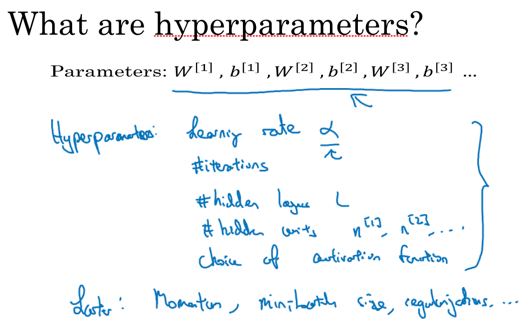Andrew老师最近创办了deeplearning.ai，主页上目前的内容是关于deep learning的课程。课程内容还是放在了Coursera - Andrew Ng - Leep Learning，所以继续学习，记录，总结。

## Why Deep Learning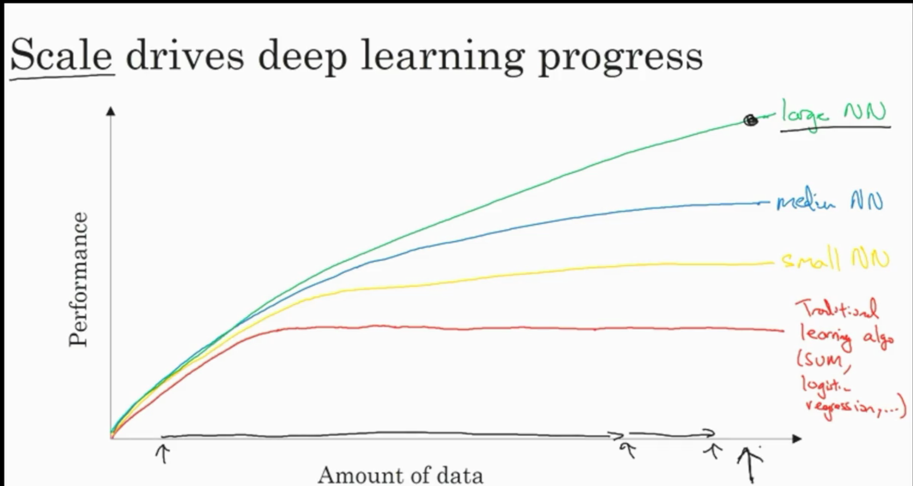## Concept

• 神经网络模型中将常量(bias node)从参数中去掉而使用新参数$b$，inter-spectrum。
• 单个误差函数记做$\mathcal{L}$，lost function，损失函数。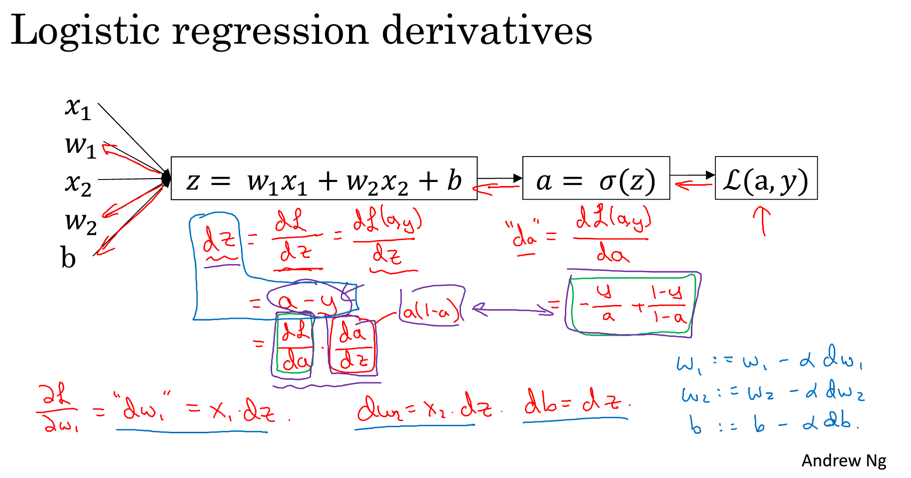$da={d\mathcal{L} \over da}=-{y\over a}+{1-y \over 1-a}$

${da \over dz}=a(1-a)$

$dz={d\mathcal{L} \over da}{da \over dz}=a-y$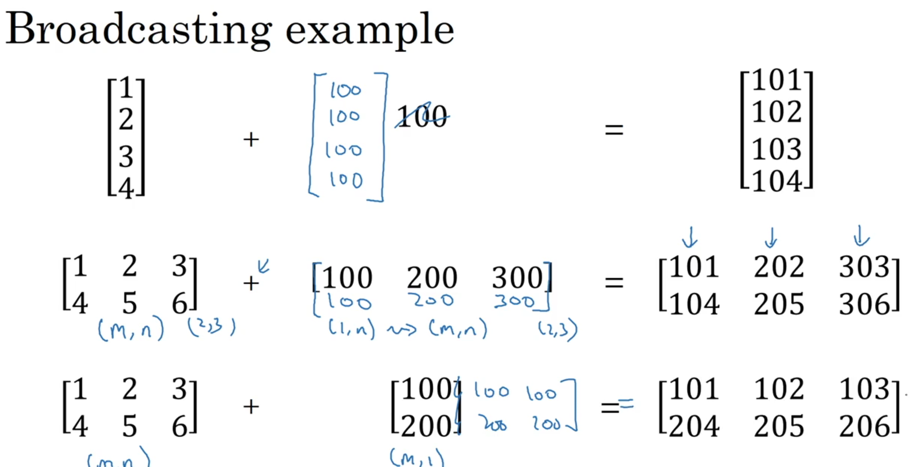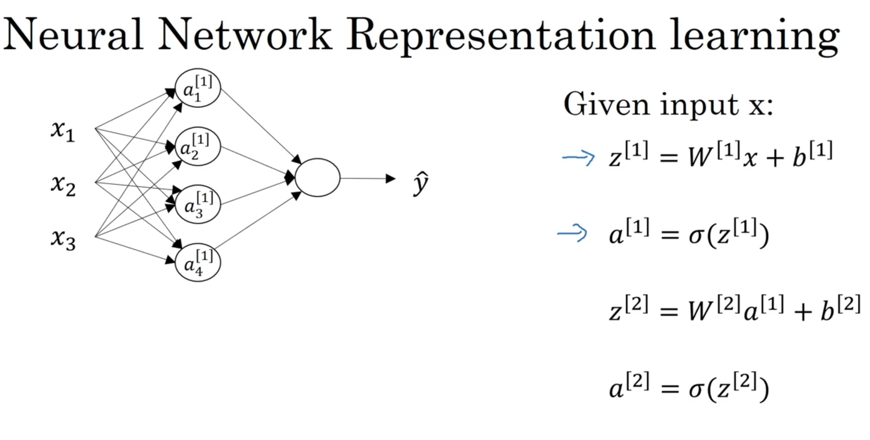activation function有几种形式：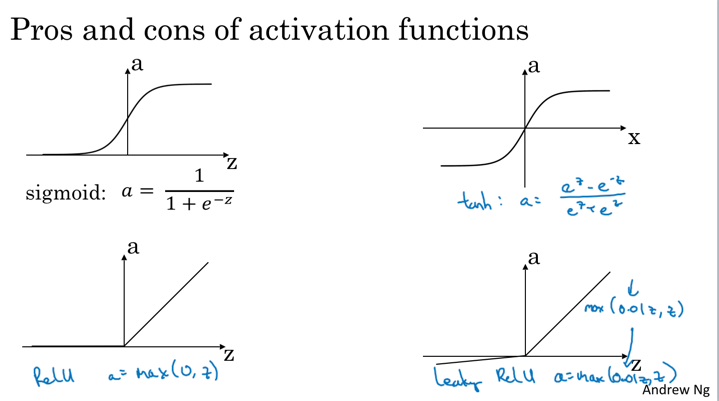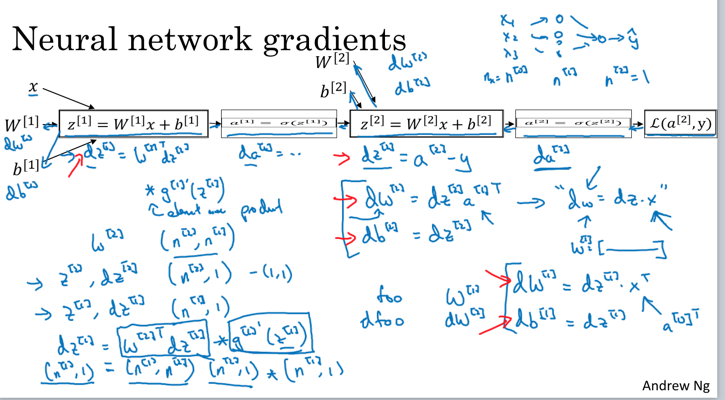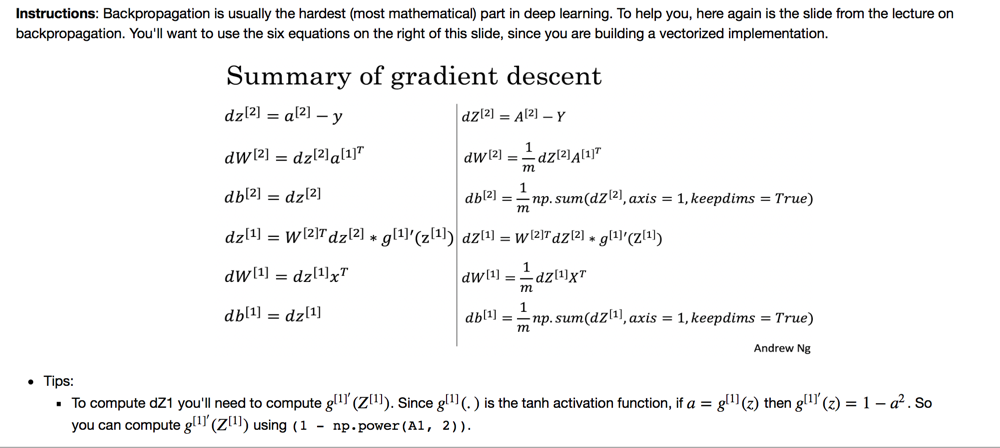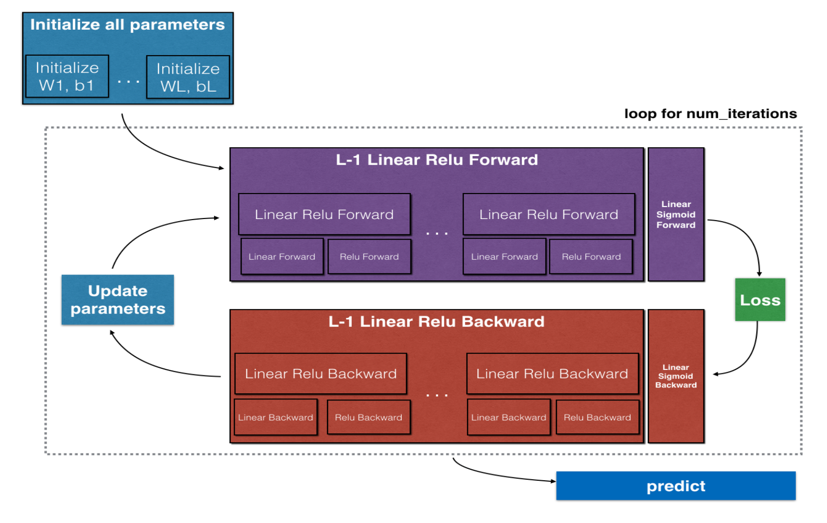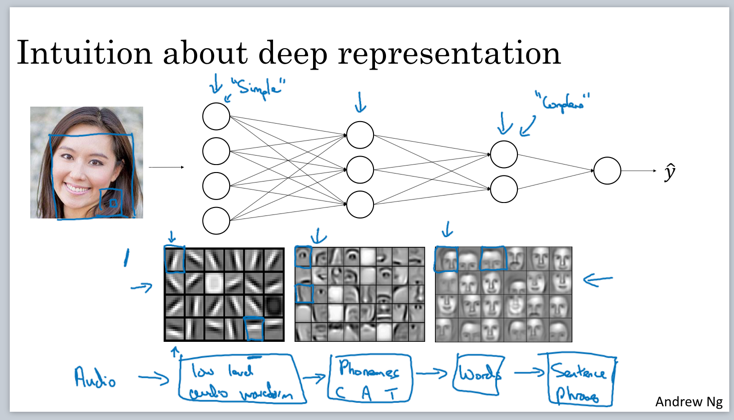hyperparameters vs parameters